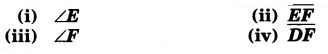# Class 7 Maths NCERT Solutions for Chapter – 7 Congruence of Triangles Ex – 7.1

## Congruence of Triangles

Question 1.
Complete the following statements :

(a) Two line segments are congruent if
(b) Among two congruent angles, one has a measure of 70°; the measure of the other angle is
(c) When we write ∠A = ∠B, we actually mean

Solution:

(a) they have the same length
(b) 70°
(c) m∠A = m∠B

Question 2.
Give any two real-life examples for congruent shapes.

Solution:

1. Two coins or notes of the same denomination.
2. Two keys of the same lock.

Question 3.
If ∆ABC = FED under the correspondence ABC ↔ FED, write all the corresponding congruent parts of the triangles.

Solution:
∆ABC = ∆FED means that ∆ABC superposes on ∆FED exactly such that the vertices of ∆ABC fall on the vertices of ∆FED in the following order A ↔ F, B ↔ E and C ↔ D.Question 4.
If ∆DEF = BCA, write the part(s) of ∆BCA that correspond toSolution:

#### Class 7 Maths NCERT Solutions for Chapter – 7 Congruence of Triangles Ex – 7.2

Lorem ipsum dolor sit amet, consectetur adipiscing elit. Phasellus cursus rutrum est nec suscipit. Ut et ultrices nisi. Vivamus id nisl ligula. Nulla sed iaculis ipsum.

Company Name